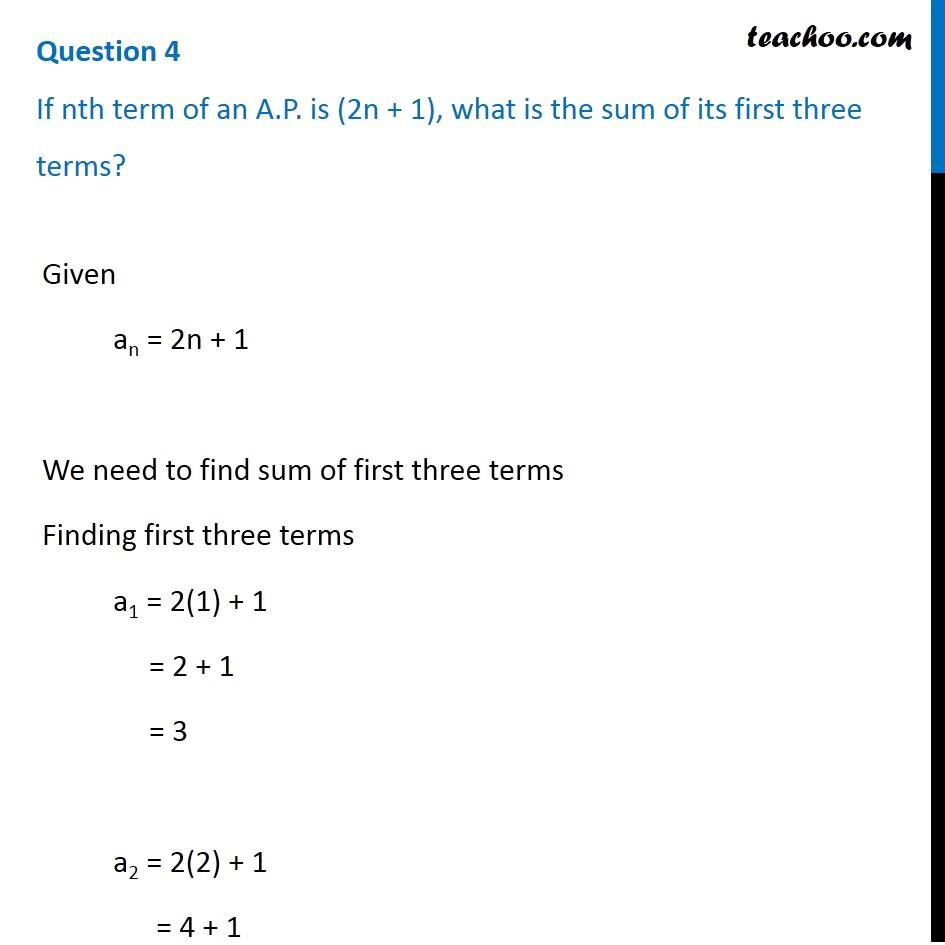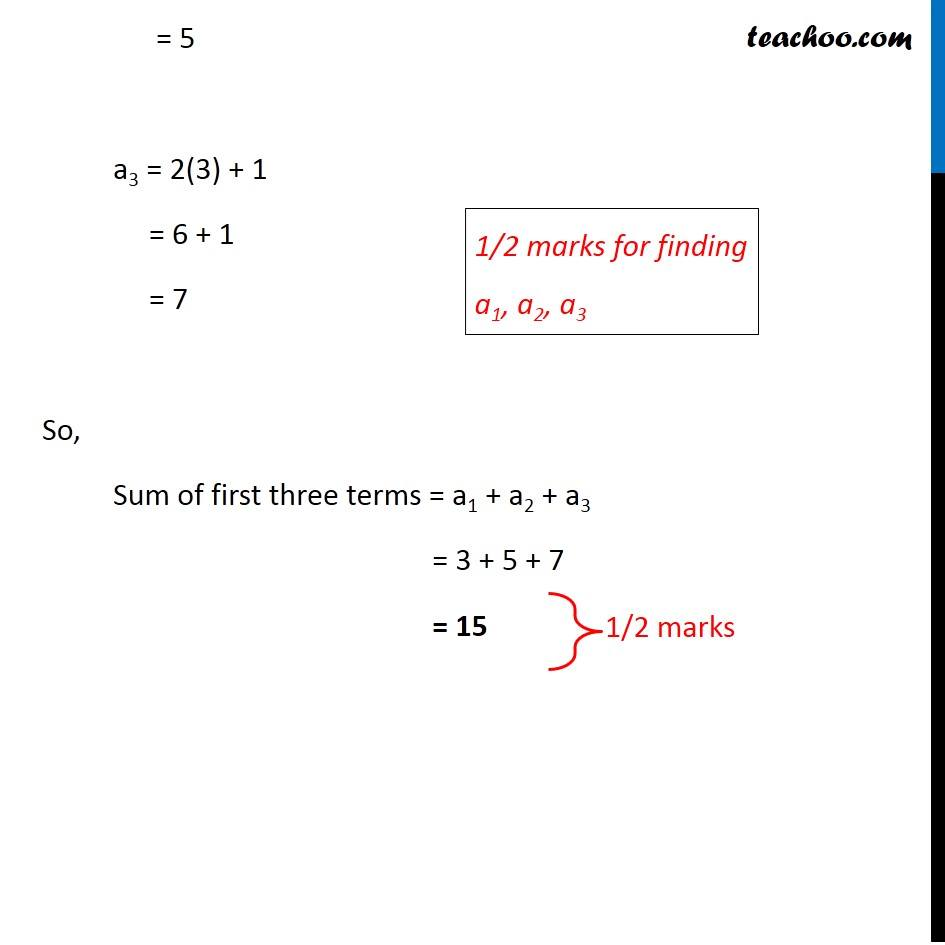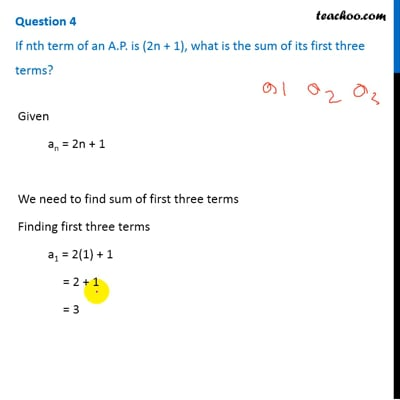CBSE Class 10 Sample Paper for 2019 Boards

Class 10
Solutions of Sample Papers for Class 10 Boards

Question 4

If n th term of an A.P. is (2n + 1), what is the sum of its first three terms?This video is only available for Teachoo black users

### Transcript

Question 4 If nth term of an A.P. is (2n + 1), what is the sum of its first three terms? Given an = 2n + 1 We need to find sum of first three terms Finding first three terms a1 = 2(1) + 1 = 2 + 1 = 3 a2 = 2(2) + 1 = 4 + 1 = 5 a3 = 2(3) + 1 = 6 + 1 = 7 1/2 marks for finding a1, a2, a3 So, Sum of first three terms = a1 + a2 + a3 = 3 + 5 + 7 = 15